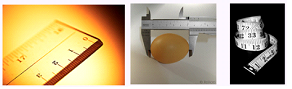##### KAPEC GEOTASK?
• The learning process is entertaining and exciting
• The solved tasks turn into coordinates
• The chance to follow your progress
• Along with your schoolmates and friends, go on an adventure, searching for hideouts
• E-mail:

# 287.3. Measurement.

#### With which device can determine length with the most precision?A.                                  B.                    C.

#### Next task: 286. Measurement.

Grade:
8
Subject
Physics
Theme
What is learned in physics?
Subtheme
Measurement.

#### Solve tasks to find hidden Geo point coordinates

1. Physics: Research of movement.
2. Algebra: Median
3. Geometry: Polygon angle sum, convex or concaved quadrangle, polygon diagonale.
4. Physics: Amount of heat.
5. Chemistry: Gas volume calculations.
6. Physics: Archimedes law.
7. Physics: Light reflection and mirrors.
8. Geometry: Parallelogram, elements. Properties and signs.
9. Geometry: Triangle in a circle, outlined circle lines center location.
10. Physics: Measurement.